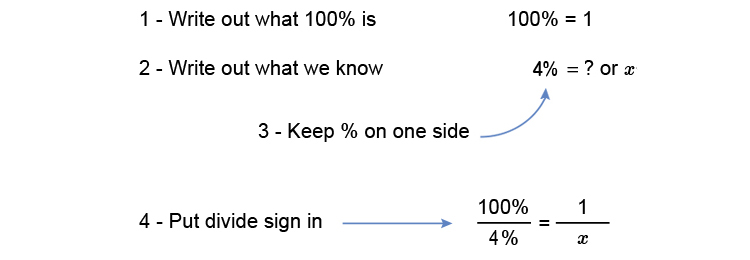# Percentages to fractions

Change 4% to a fractionMultiply both sides by x to get x on its own. (What you do to one side do to the other).

(100timesx)/4=(1timescancelx)/cancelx

(100x)/4=1

Multiply both sides by 4 to get 4 on its own.

(cancel4times100x)/cancel4=1times4

100x=4

Divide both sides by 100 to get x on its own.

(cancel100x)/cancel100=4/100

x=4/100

Simplify this to

x=1/25

Answer: x=1/25